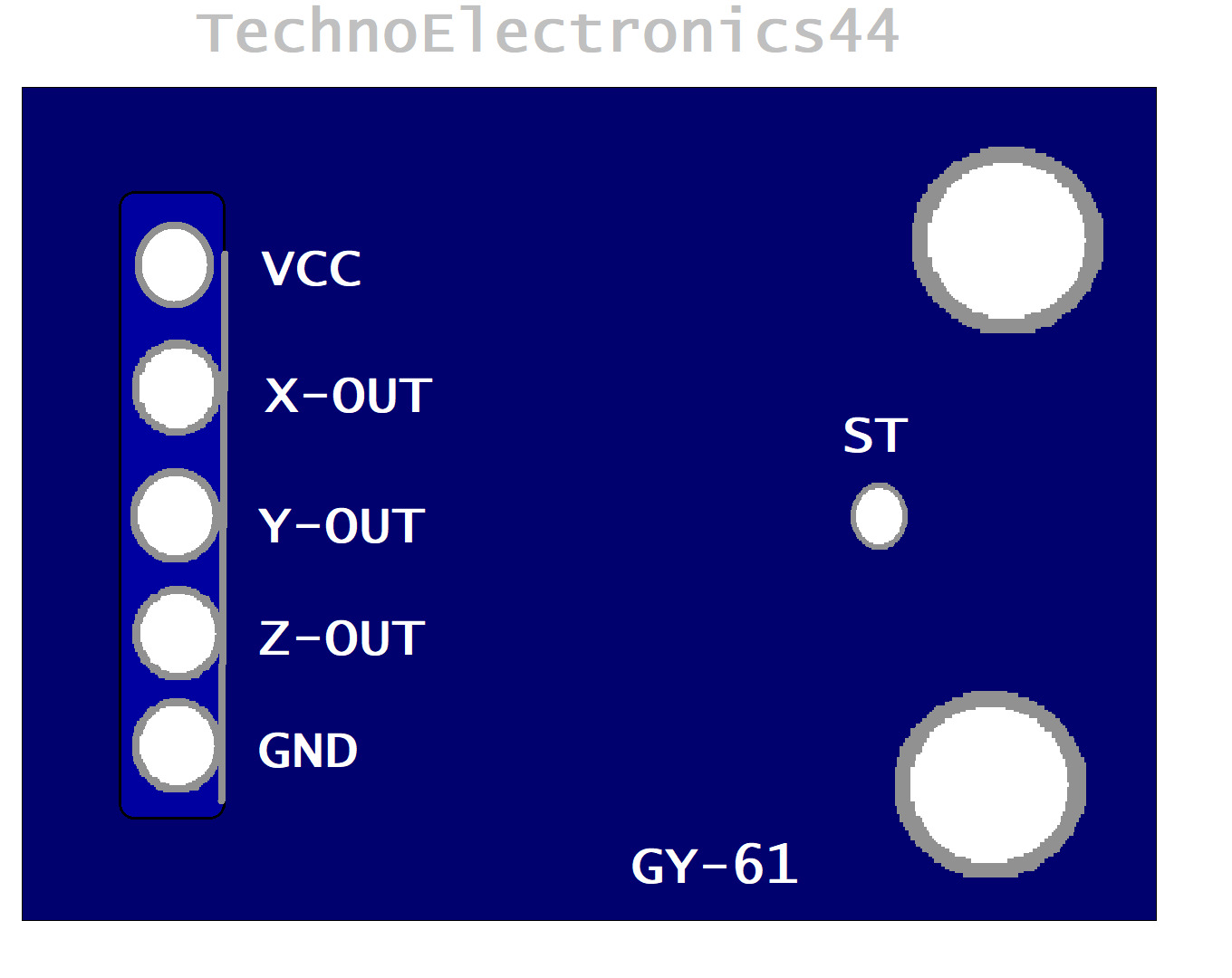## ADXL335 Accelerometer Module| Code | Circuit | Pin configuration

ADXL335 is an analog sensor and also called a triple-axis accelerometer. This device can measure the acceleration with a minimum of a full-scale of ±3g. It can measure the static acceleration in angular tilt sensing applications and dynamic acceleration in shock, motion, vibrations. It is compatible with Microcontrollers. It is designed with the structure of the MEMS technology, scroll down for MEMS technology.

Output: Analog signal

Other names: Accelerometer, MEMS, Vibrator sensor, GY-61

What is g | g-force

It is the measurement  of the force per unit mass. “g-force” of 1-g equal to the conventional value of gravitational acceleration on Earth, 1-g force is equal to 9.8 m/s2.

MEMS | what is MEMS

MEMS-Micro Electro-Mechanical System is designed with a micromachined structure built on a silicon wafer. This structure is designed with poly-silicon springs, that allows to make internal deflections at the time of acceleration is applied on the axis. The change in deflections will affect the internal capacitances between the plates that lead to change in output voltage.

So this process of change in capacitance will convert into analog output voltage at x-axis, y-axis, z-axis.

Pin Configuration

 Vcc Supply voltage positive pin GND Supply voltage negative pin X-out Analog output(X-Axis) Y-out Analog output(Y-Axis) Z-out Analog output(Z-Axis) ST Self test pinADXL335-PinoutADXL335-Pinout

Features

• Output: Analog
• Type: 3-axis
• Inbuilt voltage regulator- 3v3

Technical specifications

Operating voltage(Vs): 1.8v to 3.6v

Supply-current: 350uA at 3v

Turn ON time: 1ms

Operating temperature range: -40 ̊C to +85 ̊C.

Measurement range: ±3g

Sensitivity: 300mv/g at g=0, Vs=3v, T=25̊̊ C

Output voltage: 1.5v at g=0, Vs=3V.

For more details refer to Datasheet , you will find below

Factors affect the Output voltage

The analog output voltage depends on the three factors

Supply voltage

G-vale (up to ±3g)

Noise factor.

Supply voltage

The output voltage is proportional to the supply voltage. At Vs=3.6v the output sensitivity is 360mV/g, at Vs=2v the output sensitivity is 195mV/g.

g-value

At 0-g the output voltage is equal to Vs/2 at all supply voltages as per Datasheet.

Noise factor

The noise density decreases as supply voltage increases, At VS = 3.6 V, the X-axis and Y-axis noise density is typically 120 μg/√Hz, and VS = 2 V, the X-axis and Y-axis noise density is typically 270 μg/√Hz.

Arduino with ADXL335 | Arduino with MEMS-Accelerometer

ADXL335 is compatible with microcontroller and it provides the analog output, for calibrating the analog data we have to use the ADC-analog to digital converter. Arduino has an inbuilt 10bit-ADC it provides the minimum value is ‘0’ and the maximum value is 1023 so Arduino ADC provides 1024 discrete numbers.

Arduino Uno has 6-channel ADC from A0-A5 and ADXL335 has 3-analog outputs (X-axis, Y-axis, Z-axis).In our design, we require 3-ADC channels, 5V, GND pins of Arduino.

 ADXL335 ARDUINO UNO Vcc 5v GND GND X-out A0 y-out A1 Z-out A2 ST No connection/GND

Note : Here Vcc is connected to 5V because it has an inbuilt voltage regulator-3v3 , ADSL335 operating voltage is 3.3v.

Warning: For self-test purpose you have to connect at Vs (1.8v-3.6v), if the voltage at self-test pin crosses 3.6v it may cause to damage the device

Circuit diagramARDUINO WITH-ADXL335

## Circuit Working

From the above circuit, we can conclude that x-axis, y-axis, the z-axis is connected to analog pins of Arduino, the voltage at the analog pins(A0,A1,A2) will be converted into readings by using analog Read(x_axis) gives the readings at A0(x_out), analog Read(y_axis) gives the readings at A1(y_out),  analog Read(z_axis) gives the readings at A2(z_out). These readings are printed on the Serial monitor using “Serial.print”.

## Applications

• Mobile devices
• Gaming systems
• Disk drive protection
• Image stabilization
• Sports and health devices

PIR-Sensor

RGB-Color Sensor

IR-Sensor Module